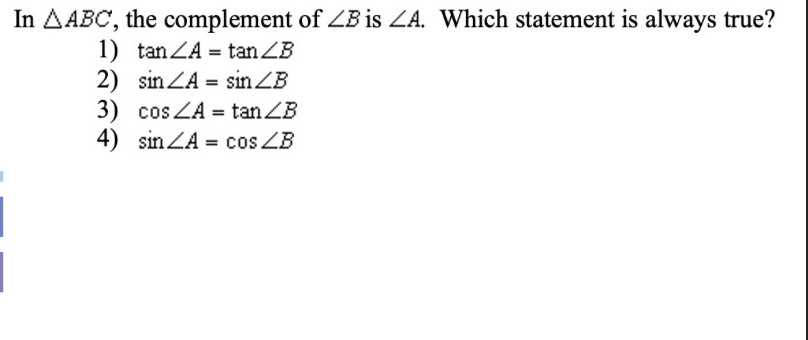### Still have math questions?

Trigonometry
QuestionIn $$\triangle A B C$$ , the complement of $$\angle B$$ is $$\angle A$$ . Which statement is always true? 1) $$\tan \angle A = \tan \angle B$$

2) $$\sin \angle A = \sin \angle B$$

3) $$\cos \angle A = \tan \angle B$$

4) $$\sin \angle A = \cos \angle B$$